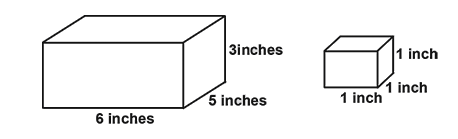QuestionAnswers

# How many 1 inch cubes will completely fill the carton shown?Verified
128.4k+ views
Hint: This is the formula for the volume of a cuboidal box
$Volume{\text{ }} = {\text{ }}length \times width\; \times height$
Volume of a cubical box can be found out by
$Volume{\text{ }} = {\text{ }}length \times length\; \times length$

Complete step by step solution: Start by putting a layer of blocks on the bottom of the box. Since the box is $6$ inches long you can place $6$ blocks along the front edge of the box. Then you can place a second row of $5$ blocks behind it and again another row of $5$ blocks behind the second row. You have placed $6 \times \;5{\text{ }} = {\text{ }}30$ blocks on the bottom layer of the box.
The box is $3$ inches high so you can put a second layer of $30$ blocks on top of the first layer and then a third layer of $30$ blocks to fill the box. Hence you have filled the box with $3 \times \;30{\text{ }} = {\text{ }}90$, one cubic inch blocks.
Hence the volume of the box is $36$ cubic inches and you obtained the number $90$ from $6 \times \;5 \times 3{\text{ }} = {\text{ }}90$ that is the length times the width times the height.
Also, we can use the formula $Volume{\text{ }} = {\text{ }}length \times width\; \times height$
to calculate the volume of the box, which is $90$

Note: Carton of length $6$ inch- $6$ number of $1$ inch cube can come.
Width= $5$ inch- $5$ cube will fill. Height=$3$ inch- $3$ cube will fill.
So, total number of cubes is$6 \times \;5 \times 3{\text{ }} = {\text{ }}90$
The number of One-inch cubes that can be filled in a bigger one can be calculated just by dividing the volume of the bigger box with the smaller one.